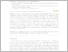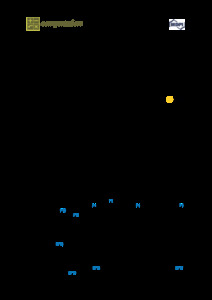Repository: Freie Universität Berlin, Math Department

# Singularly perturbed forward-backward stochastic differential equations: application to the optimal control of bilinear systems

Kebiri, O. and Neureither, L. and Hartmann, C. (2018) Singularly perturbed forward-backward stochastic differential equations: application to the optimal control of bilinear systems. Computation, 6 (3). p. 41. ISSN 2079-3197 (online)PDF
Restricted to Registered users only

365kBPreview
PDF - Published Version
327kB

Official URL: https://dx.doi.org/10.3390/computation6030041

## Abstract

We study linear-quadratic stochastic optimal control problems with bilinear state dependence for which the underlying stochastic differential equation (SDE) consists of slow and fast degrees of freedom. We show that, in the same way in which the underlying dynamics can be well approximated by a reduced order effective dynamics in the time scale limit (using classical homogenziation results), the associated optimal expected cost converges in the time scale limit to an effective optimal cost. This entails that we can well approximate the stochastic optimal control for the whole system by the reduced order stochastic optimal control, which is clearly easier to solve because of lower dimensionality. The approach uses an equivalent formulation of the Hamilton-Jacobi-Bellman (HJB) equation, in terms of forward-backward SDEs (FBSDEs). We exploit the efficient solvability of FBSDEs via a least squares Monte Carlo algorithm and show its applicability by a suitable numerical example.

Item Type: Article SFB 1114 Preprint in SciRate.arXiv:1802.04978 Mathematical and Computer Sciences > Mathematics > Applied Mathematics Department of Mathematics and Computer Science > Institute of Mathematics 2222 Silvia Hoemke 16 Feb 2018 15:50 16 Jan 2020 11:39

Repository Staff Only: item control page